# Telling time to the nearest minute: labeled clock | Math | 3rd grade | Khan Academy

##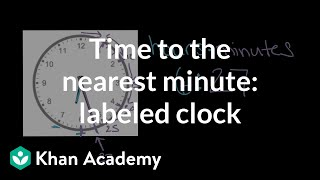By Skubes ed

problems involving addition and subtraction of time intervals in minutes, e.g., by representing the problem on a number line diagram.# Elapsed Time Word Problems

##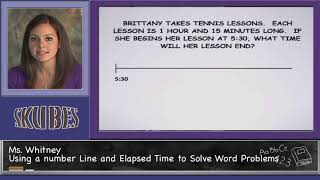By Skubes ed

problems involving addition and subtraction of time intervals in minutes, e.g., by representing the problem on a number line diagram.# Algebra for 4th Graders:CCSSM 4.NF.3d

##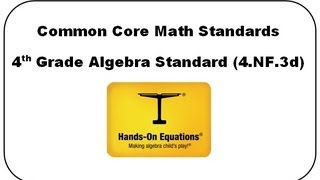By Henry Borenson

Use visual models and equations to represent and solve word problems involving addition and subtraction of fractions (4.NF.3d)# [4.NF.3d-1.0] Word Problems: Addition - Common Core Standard

##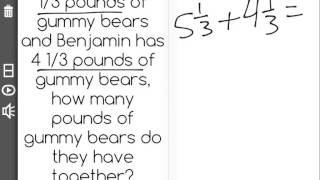By Freckle education

Solve word problems involving addition and subtraction of fractions referring to the same whole and having like denominators, e.g., by using visual fractions models and equations to represent to problem.# Telling Time to the Nearest Minute

##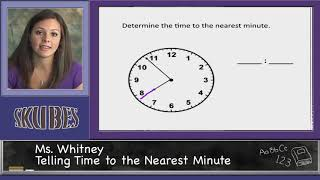By Skubes ed

Tell and write time to the nearest minute and measure time intervals in minutes. Solve word problems involving addition and subtraction of time intervals in minutes, e.g., by representing the problem on a number line diagram.# Multi-step word problem with decimals and subtraction

##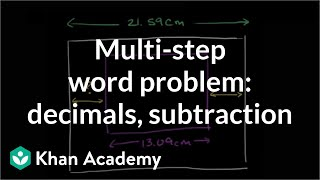By Khan Academy

First visualize this word problem then use subtraction and multiplication of decimals and fractions to get at the answer.# Addition and subtraction with significant figures | Decimals | Pre-Algebra | Khan Academy

##By Khan Academy

This video shows us the rules for adding and subtracting measurements to show our level of accuracy. Note that the rules for addition/subtraction with significant figures are VERY different than the rules for mulitplication/division. Think about which number is the most precise!# Multi-step word problem with decimals and subtraction

##By Khan,Academy

First visualize this word problem then use subtraction and multiplication of decimals and fractions to get at the answer.##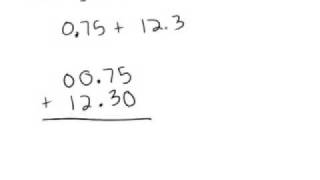By TeacherTube Math

This video is from the TeacherTube website and the instructor shows how to add and subtract decimals. She shows how to line up the decimal points in the numbers. Instructor appears to be using computer software for demonstration.# [4.NF.3d-1.0] Word Problems: Addition - Common Core Standard

##By Freckle by Renaissance

Solve word problems involving addition and subtraction of fractions referring to the same whole and having like denominators, e.g., by using visual fractions models and equations to represent to problem. Front Row is a free, adaptive, Common Core aligned math program for teachers and students in kindergarten through eighth grade. Front Row allows students to practice math at their own pace - learning advanced concepts when they're ready and receiving remediation when they struggle. Front Row provides teachers with access to a detailed data dashboard and weekly email reports that show which standards are causing students difficulty, what small groups can be formed for interventions, and how their students are progressing in math.# One-step addition and subtraction equations with fractions and decimals

##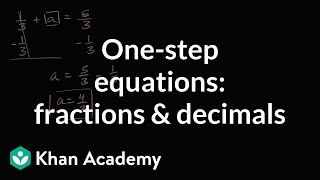By Khan Academy

Learn how to solve one-step addition and subtraction equations that have fractions and decimals in them.# One-step addition and subtraction equations with fractions and decimals

##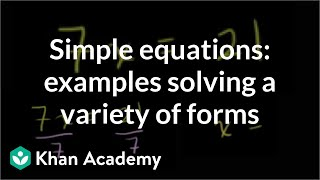By Khan Academy

Some quick examples to practice solving a variety of one step equations. All 4 operations (add, subtract, multiple, divide) are paired with variables.# One-step addition and subtraction equations with fractions and decimals

##By Khan Academy

Learn how to solve one-step addition and subtraction equations by adding or subtracting the same thing from both sides of the equation.# One-step addition and subtraction equations with fractions and decimals

##By Khan Academy

Learn how to solve this equation: a + 5 = 54ï¿½ï¿½ï¿½ï¿½ï¿½ï¿½ï¿½ï¿½ï¿½ï¿½ï¿½ï¿½##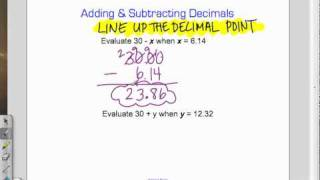By Mathademics# division of decimals- modeling

##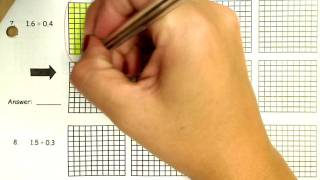By nsvolley28# Adding and subtracting decimals word problems

##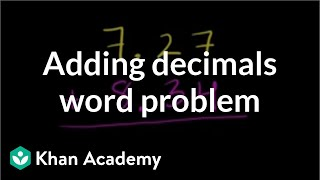By Khan Academy

Here's a simple word problem asking us to put our adding decimals skill to work. We sound like a broken record, but be sure and ALIGN DECIMALS.# Adding and subtracting decimals word problems

##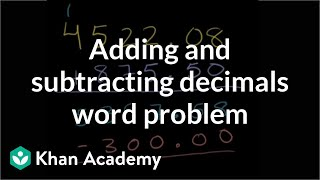By Khan Academy

Let's get fancy and practice adding and subtracting decimals in the same word problem.# Subtracting Decimals - Word Problem

##By Khan Academy

This video is from Sal Khan about subtracting decimals using the problem: Leo has \$4 522.08 in his bank account. He deposits another \$875.50 and then withdraws \$300.00 in cash. How much is left in his bank account? Mr. Khan uses computer software for demonstration. The screen is a little 'busy' so the viewer may want to open the screen to 'full screen' to see all more clearly.# Subtracting decimals

##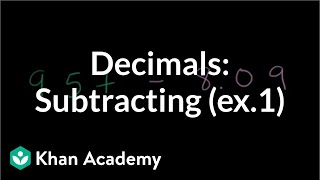By Khan Academy

Subtracting decimals...up to the hundredths placement. Line up those decimals before you start.